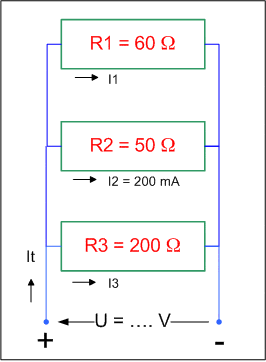# Very simple question law of ohm

n124122*U=voltage

U=IR (V=IR)

## The Attempt at a Solution

200mA=0,2A --> 0,2*50=10V...? So because it's parallel the source should be 10V too right?

Homework Helper*U=voltage

U=IR (V=IR)

## The Attempt at a Solution

200mA=0,2A --> 0,2*50=10V...? So because it's parallel the source should be 10V too right?
Right. :)